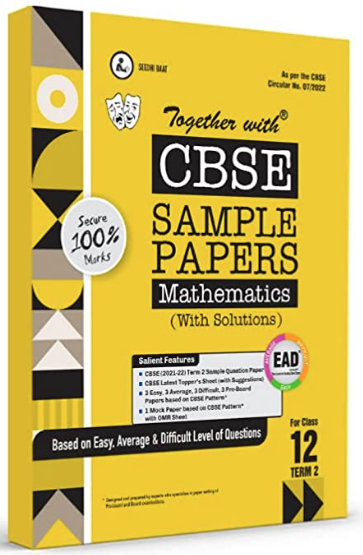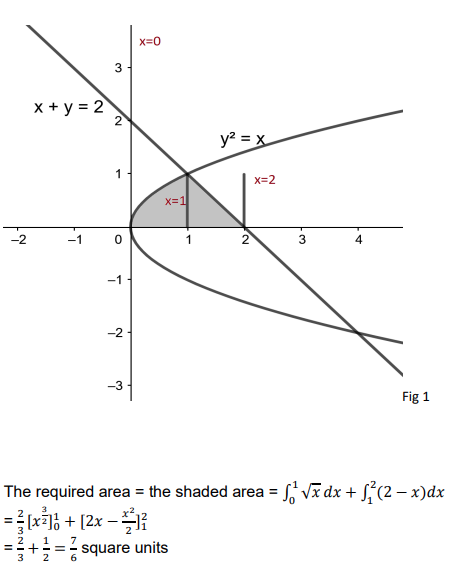# Using integration, find the area of the region in the first quadrant enclosed by the line x + y = 2, the parabola y 2 = x and the x-axis.

CBSE Sample Question Paper, Class 12 Mathematics Term 2 Question - Using integration, find the area of the region in the first quadrant enclosed by the line x + y = 2, the parabola y 2 = x and the x-axis.## Using integration, find the area of the region in the first quadrant enclosed by the line x + y = 2, the parabola y 2 = x and the x-axis.